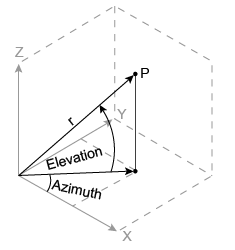# cart2sph

Transform Cartesian coordinates to spherical

## Syntax

``````[azimuth,elevation,r] = cart2sph(x,y,z)``````

## Description

example

``````[azimuth,elevation,r] = cart2sph(x,y,z)``` transforms corresponding elements of the Cartesian coordinate arrays `x`, `y`, and `z` to spherical coordinates `azimuth`, `elevation`, and `r`.```

## Examples

collapse all

Convert the Cartesian coordinates defined by corresponding entries in the matrices `x`, `y`, and `z` to spherical coordinates `az`, `el`, and `r`. These points correspond to the eight vertices of a cube.

`x = [1 1 1 1; -1 -1 -1 -1]`
```x = 2×4 1 1 1 1 -1 -1 -1 -1 ```
`y = [1 1 -1 -1; 1 1 -1 -1]`
```y = 2×4 1 1 -1 -1 1 1 -1 -1 ```
`z = [1 -1 1 -1; 1 -1 1 -1]`
```z = 2×4 1 -1 1 -1 1 -1 1 -1 ```
`[az,el,r] = cart2sph(x,y,z)`
```az = 2×4 0.7854 0.7854 -0.7854 -0.7854 2.3562 2.3562 -2.3562 -2.3562 ```
```el = 2×4 0.6155 -0.6155 0.6155 -0.6155 0.6155 -0.6155 0.6155 -0.6155 ```
```r = 2×4 1.7321 1.7321 1.7321 1.7321 1.7321 1.7321 1.7321 1.7321 ```

## Input Arguments

collapse all

Cartesian coordinates, specified as scalars, vectors, matrices, or multidimensional arrays. `x`, `y`, and `z` must be the same size, or any of them can be scalar.

Data Types: `single` | `double`

## Output Arguments

collapse all

Azimuth angle, returned as an array. `azimuth` is the counterclockwise angle in the x-y plane measured in radians from the positive x-axis. The value of the angle is in the range ```[-pi pi]```.

Elevation angle, returned as an array. `elevation` is the elevation angle in radians from the x-y plane. The value of the angle is in the range `[-pi/2, pi/2]`.

Radius, returned as an array. `r` is the distance from the origin to a point. The length units of `r` are arbitrary, matching the units of the input arrays `x`, `y`, and `z`.

## Algorithms

The mapping from three-dimensional Cartesian coordinates to spherical coordinates is

```azimuth = atan2(y,x) elevation = atan2(z,sqrt(x.^2 + y.^2)) r = sqrt(x.^2 + y.^2 + z.^2)```The notation for spherical coordinates is not standard. For the `cart2sph` function, `elevation` is measured from the x-y plane. Notice that if ```elevation = 0```, the point is in the x-y plane. If `elevation = pi/2`, then the point is on the positive z-axis.